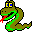#Boost C++ Libraries

...one of the most highly regarded and expertly designed C++ library projects in the world.

This is the documentation for an old version of Boost. Click here to view this page for the latest version.#Function betweenness_centrality_clustering

boost::betweenness_centrality_clustering — Graph clustering based on edge betweenness centrality.

## Synopsis

```template<typename MutableGraph, typename Done, typename EdgeCentralityMap,
typename VertexIndexMap>
void betweenness_centrality_clustering(MutableGraph & g, Done done,
EdgeCentralityMap edge_centrality,
VertexIndexMap vertex_index);
template<typename MutableGraph, typename Done, typename EdgeCentralityMap>
void betweenness_centrality_clustering(MutableGraph & g, Done done,
EdgeCentralityMap edge_centrality);
template<typename MutableGraph, typename Done>
void betweenness_centrality_clustering(MutableGraph & g, Done done);
```

## Description

This algorithm implements graph clustering based on edge betweenness centrality. It is an iterative algorithm, where in each step it compute the edge betweenness centrality (via brandes_betweenness_centrality) and removes the edge with the maximum betweenness centrality. The done function object determines when the algorithm terminates (the edge found when the algorithm terminates will not be removed).

## Parameters

IN: const Graph& g
The graph object on which the algorithm will be applied. The type Graph must be a model of Vertex List Graph and Incidence Graph. When an edge centrality map is supplied, it must also model Edge List Graph and MutableGraph.
Python: The parameter is named graph.
IN: Done done
The function object that indicates termination of the algorithm. It must be a ternary function object thats accepts the maximum centrality, the descriptor of the edge that will be removed, and the graph g.
Python: Any callable Python object will suffice.
OUT/UTIL: EdgeCentralityMap edge_centrality_map
This property map is used to accumulate the betweenness centrality of each edge, and is a secondary form of output for the algorithm. The type EdgeCentralityMap must be a model of Read/Write Property Map, with the graph's edge descriptor type as its key type. The value type of this property map should be the same as the value type of the CentralityMap property map.
Default: a dummy_property_map, which requires no work to compute and returns no answer.
Python: The color map must be a edge_double_map for the graph.
Python default: graph.get_edge_double_map("centrality")
IN: VertexIndexMap vertex_index
This maps each vertex to an integer in the range [0, num_vertices(g)). This is necessary for efficient updates of the heap data structure when an edge is relaxed. The type VertexIndexMap must be a model of Readable Property Map. The value type of the map must be an integer type. The vertex descriptor type of the graph needs to be usable as the key type of the map.
Default: get(vertex_index, g). Note: if you use this default, make sure your graph has an internal vertex_index property. For example, adjacenty_list with VertexList=listS does not have an internal vertex_index property.
Python: Unsupported parameter.

### Where Defined

<boost/graph/bc_clustering.hpp>
 Copyright © 2004 Douglas Gregor, Indiana University (dgregor@cs.indiana.edu) Andrew Lumsdaine, Indiana University (lums@osl.iu.edu)